# CS5625 PA2 Textures and Articulated Characters

Out: Thursday February 26, 2015

Due: Thursday March 12, 2015 at 11:59pm

Work in groups of 2.

## Overview

This is a long assignment with many tasks on rather unrelated topics. You will also surely face unexpected bugs. Start early.

In this programming assignment, you will implement:

1. a shading model using a tangent-space normal map,
2. a shading model for a perfect mirror material taking a cube map as the source of incoming light,
3. a system for rendering the scene into a cube map so that it can be used with the shading model in the previous item,
4. a post-processing pass that implements the "bloom" effect,
5. blend shapes for facial expressions, and
6. linear blend skinning for articulated character animations.

Not counting Task 1 which we ask you to port your old code, this assignment has 7 tasks which can be divide them into 4 groups which are more or less independent of one another:

2. Task 3, 4, and 5
As a result, you do not have to complete the tasks in the order specified in this document.

We will be reusing many forward shaders from PA1. Edit:

so that they contain the appropriate part of your solution code from PA1. You should copy your Blinn-Phong shader implementation into the shader normalmap_blinnphong.frag as a starting point for Task 2. You might remember from PA1 that was ask you to modify the forward renderer too. However, in this assignment, its interface changed slightly, and we don't want to burden you with plumbing the data through again.

Before starting on the assignment, if you run the cs5625.pa2.PA2_Textures class, you will see the following rendering: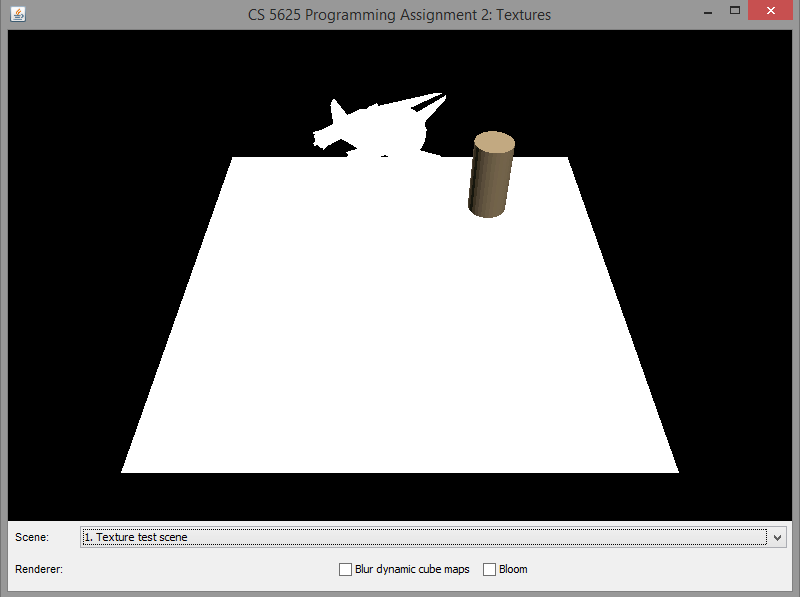We have provided three scenes to test your code. The first is the "texture test" scene that contains new features we will asks you to implement in next tasks. The last two are the "default" and the "material test" scenes from the last assignment. After porting your code, the image of the texture test scene should become: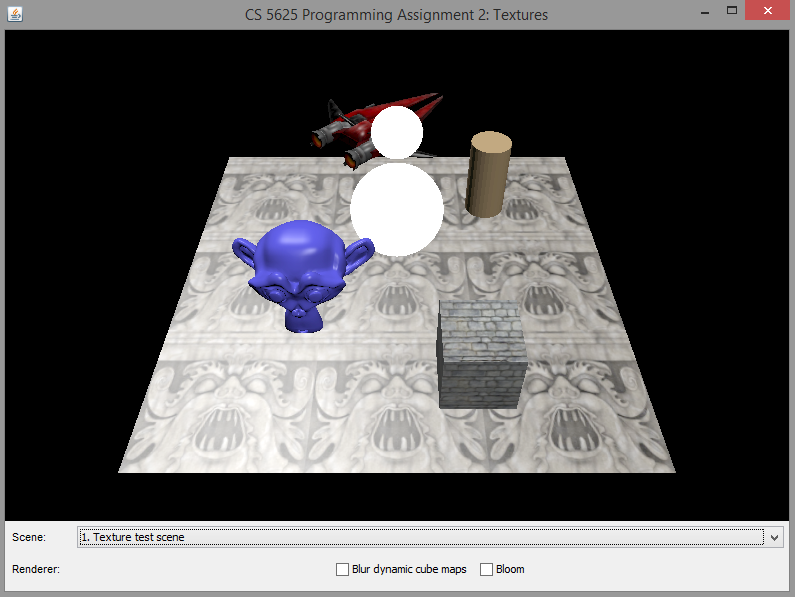Note that the floor and the two spheres in the center are still left white. This is because they have new materials that you will be implementing. For the other two scenes, you should be able to see the same results that you produced in PA1.

## Task 2: Tangent-space normal mapping

Edit:

so that it implements a Blinn-Phong shading model together with a tangent space normal map. The only difference between this model and the standard version is how the normal at the shaded point is computed. In the standard version, we just normalize the vector given to us through the varying variable geom_normal. In this version, however, we first need to compute an orthonormal tangent space at the shaded point and then use it, together with the texture value of the normal map, to compute the effective normal.

Let us first discuss the computation of the tangent space. The vertex shader will pass three varying variables: geom_normal, geom_tangent, and geom_bitangent to the fragment shader. The vectors contained in these variabled are interpolated from those same vectors at the vertices. Hence, they are not necessarily orthonormal or even normalized. To recover, an orthonomal frame ($\mathbf{t}$, $\mathbf{b}$, $\mathbf{n}$), we suggest that you:

1. Normalize the geom_normal variable to get the normal vector $\mathbf{n}$.
2. Project geom_tangent to the plane perpendicular to $\mathbf{n}$ and then normalize it to get $\mathbf{t}$.
3. Compute the cross product $\tilde{\mathbf{b}} = \mathbf{n} \times \mathbf{t}$.
4. If the dot product between geom_bitangent and $\tilde{\mathbf{b}}$ is greater than 0, set $\mathbf{b} = \tilde{\mathbf{b}}$. Otherwise, set $\mathbf{b} = -\tilde{\mathbf{b}}$.
In other words, we want a frame where the normal is parallel to the interpolated value. The tangent vector is as close as possible to the interpolated value but perpendicular to the normal. The bitangent is "redefined" to be parallel to the cross product between $\mathbf{n}$ and $\mathbf{t}$, but pointing in the direction that preserves the handedness given by the original mesh data.

Next is the computation of the effective normal. The normal map encodes the normal texture as a color as follows: $$\begin{bmatrix} r \\ g \\ b\end{bmatrix} = \begin{bmatrix} (\bar{\mathbf{n}}_x + 1) /2 \\ (\bar{\mathbf{n}}_y + 1) /2 \\ (\bar{\mathbf{n}}_z + 1) /2 \end{bmatrix}$$ where $\bar{\mathbf{n}} = (\bar{\mathbf{n}}_x, \bar{\mathbf{n}}_y, \bar{\mathbf{n}}_z)^T$, in tangent space, being encoded. You should recover the tangent space normal from the color of the texture at the shaded point. Then, the effective normal $\mathbf{n}_{\mathrm{eff}}$ is given by: $$\mathbf{n}_{\mathrm{eff}} = \bar{\mathbf{n}}_x \mathbf{t} + \bar{\mathbf{n}}_y \mathbf{b} + \bar{\mathbf{n}}_z \mathbf{n}.$$ Proceed by using the effective normal to shade the fragment.

After implementing the shader, the floor and the cube in the "texture test" scene should become much more interesting:

Noticde the differences between the last image of Task 1 and the previous images. You can see that the ground and the cube appears to have much more surface details.

## Task 3: Reflective material from cube map

Edit:

so that they together implement a material that reflects an environment map, represented by a cube map, like a perfect mirror.

The ReflectionMaterial material class contains two important fields:

• cubeMap, which represents the environment map, and
• worldToCubeMap, which is a 3x3 matrix that transforms a direction from world space to cube map space. We shall refer to this matrix as $M_{\mathrm{world}\rightarrow\mathrm{cube}}$. This matrix is used so that different instances of the material can reflect a differently "rotated" version of the cube map.

Let us first discuss how the reflective material works. Suppose, at the shaded point, we have a computed the direction the direction $\mathbf{r}_{\mathrm{cube}}$, which represents the direction that a perfect mirror should reflect the view direction from its surface. Then, the fragment color of the shaded point is simply given by:

gl_FragColor = textureCube(mat_cubeMap, $\mathbf{r}_{\mathrm{cube}}$);

How do we get $\mathbf{r}_{\mathrm{cube}}$? The fragment shader is given two varying variables geom_normal and geom_position, so we can compute a view direction and a normal vector from it. Since these uniforms are in camera space, the resulting vectors are also in camera space, which we shall denote by $\mathbf{v}_{\mathrm{cam}}$ and $\mathbf{n}_{\mathrm{cam}}$. From these vectors, we can compute the reflected direction $\mathbf{r}_{\mathrm{cam}}$, which is also in camera space. (As a note, you can use the GLSL built-in reflect function to compute $\mathrm{r}_{\mathrm{cube}}$, but BE VERY CAREFUL OF WHAT IT EXPECTS AS ARGUMENTS.) This is not exactly what we want because we want the vector in the cube map space.

To get the vector in cube map space, first realize that, if $\mathbf{r}_{\mathrm{world}}$ is the reflected direction in world space, then $$\mathbf{r}_{\mathrm{cam}} = M_{\mathrm{view}} \mathbf{r}_{\mathrm{world}}.$$ In other words, $$\mathbf{r}_{\mathrm{world}} = M_{\mathrm{view}}^{-1} \mathbf{r}_{\mathrm{cam}}.$$ Once we have the vectors in world space, we can use $M_{\mathrm{world}\rightarrow\mathrm{cube}}$ to transform them to the cube map space: \begin{align*} \mathbf{r}_{\mathrm{cube}} &= M_{\mathrm{world}\rightarrow\mathrm{cube}} \mathbf{r}_{\mathrm{world}} = M_{\mathrm{world}\rightarrow\mathrm{cube}} M_{\mathrm{view}}^{-1} \mathbf{r}_{\mathrm{cam}}. \end{align*}

Next, let us discuss the source of the cube map. The interface TextureCubeMapData represents an object that can fulfill the functionality. It is implemented by two classes for two different situations:

• The FileTextureCubeMapData class represents a cube map that is constructed from 6 images located in storage. We will be concerned with only this class in this task.
• The RenderedTextureCubeMapData class represents a dynamic cube map that is renderered each frame. We will work with this one in the next task.
The process that derives an OpenGL cube map from these objects has already been implemented in the forward renderer, so you do not have to worry about it. Nevertheless, you are responsible for setting up the right transformations so that the cube map are looked up correctly.

A correct implementation of the cube map should produce the following appearance on the top sphere:

Note that, since we have not implemented the dynamic cube map, the bottom sphere would display some random images depending on the contents of the GPU memory before the program ran. As such, the images displayed by your program might be different from what are shown in the example images above. This is not an issue, and you should proceed to the next task.

## Task 4: Dynamic cube map

Edit:

so that it renders the scene and stores the results in cube maps that can be used as environment maps later.

How a cube map should be rendered is determined by the CubeMapProxy object, which represents an imaginary cube located in the scene. A CubeMapProxy has a name by which the RenderedTextureCubeMapData refers to it. It also has information on the resolution of the cube map and other rendering parameters.

The ForwardRenderer locates all the CubeMapProxy objects in the scene using the collectCubeMapProxies method. It stores the proxies in a HashMap called cubeMaps so that they can be indexed by name. For each proxy, it creates an auxiliary object of class CubeMapInfo that contains several objects useful for cube map rendering:

• proxy is the CubeMapProxy itself,
• node is the SceneTreeNode containing the proxy,
• cubeMapBuffers is a collection of buffers in which the cube map will be rendered into,
• textureRectBuffers is a collection of buffers made of TextureRect, having the same size as a side of the cube map.
You will see that, in the code, the renderCubeMapProxies method iterates through all the proxies, and it allocates the cubeMapBuffers and textureRectBuffers for each of the proxy.

To render a cube map, you should iterate through its six sides. For each side, set up the camera so that:

1. The camera is located at the center of the cube.
2. It looks through the correct side of the cube.
3. When setting up the perspective camera, set the near clip to the distance between the center and the side, and set the far clip to the farClip field of the CubeMapProxy object.
To do this, you will need to modify three fields of the ForwardRenderer: projectionMatrix, viewMatrix, and inverseViewMatrix. You might find that the makeProjectionMatrix and the makeLookAtMatrix methods in the VectorMathUtil class useful. Looking at the render method of the ForwardRenderer, you shall discover that you can render the whole scene to a texture rectangle by calling the renderSceneToTextureRectBufferAndSwap method. Also, the proxy resides in a scene tree node, so it has its own modeling transformation. You need to take into account this transformation when setting up the cameras.

Due to the filtering that we will be performing in the next section, we advise that you render to the textureRectBuffers first, then copy the resulting content to the appropriate side of cubeMapBuffers.

A correct implementation of the dynamic cube map rendering should produce the following images:

## Task 5: Cube map filtering

We have implemented a mechanism for dynamic cube map in the last task, which allows us to simulate a mirror-like object being embedded in the scene. One way to simulate roughness of the material's surface is to blur the cube map. In this way, the higher the blurring, the rougher the surface becomes.

Edit:

so that the renderer applies a Gaussian blur to the rendered cube map when blurring is enabled by the program.

In the last task, you should have rendered the scene to the textureRectBuffers before copying the resulting image to the appropriate cube map side. In this task, before copying the image to the cube map, you should run the Gaussian blur shader to the rendered image two times, one for the x-axis and another for the y-axis, if the dynamicCubeMapBlurringEnabled field is set to true.

The gaussian blur fragment shader should implement a 1D Gaussian blur. To get a 2D blur, you have to apply it two times. The shader contains the following uniforms that specify the Gaussian kernel:

• size is a half of the width of the window of the kernel. Namely, when performing convolution, the shader will only look at the $2\cdot \mathrm{size} + 1$ pixels (in the appropriate direction) centered at the pixel specified by geom_texCoord.
• stdev is the standard deviation ($\sigma$) of the Gaussian kernel. Its unit is "pixel width."
• axis specifies the axis to apply the Gaussian blur. The value of 0 indicates the x-axis, and the value 1 indicates the y-axis.
You should set their values so that they agree with the specification in the CubeMapProxy object. That is,
• set size to the gaussianKernelSize field of CubeMapProxy,
• set stdev to the gaussianKernelStdev field.
Lastly, axis should be set to 0 in one pass and 1 in another pass that you apply the shader.

A correct implementation should yield the following differences between the rendered images: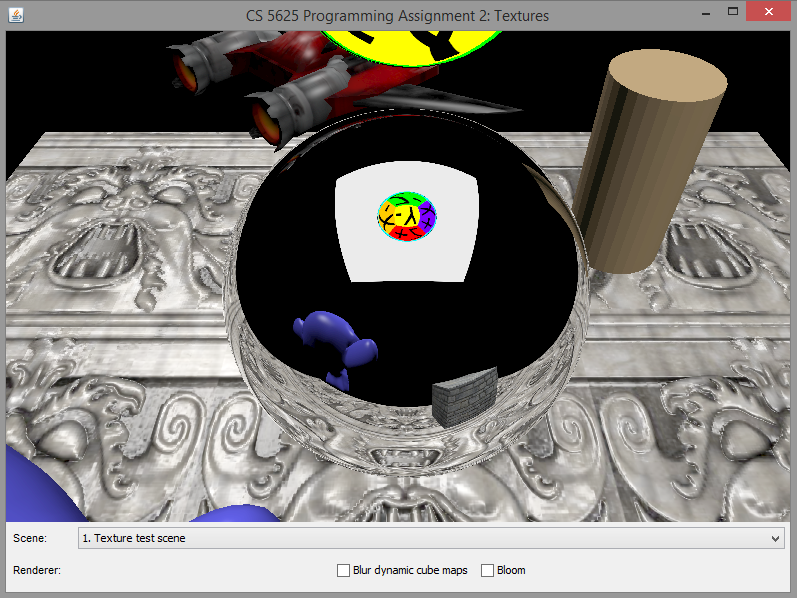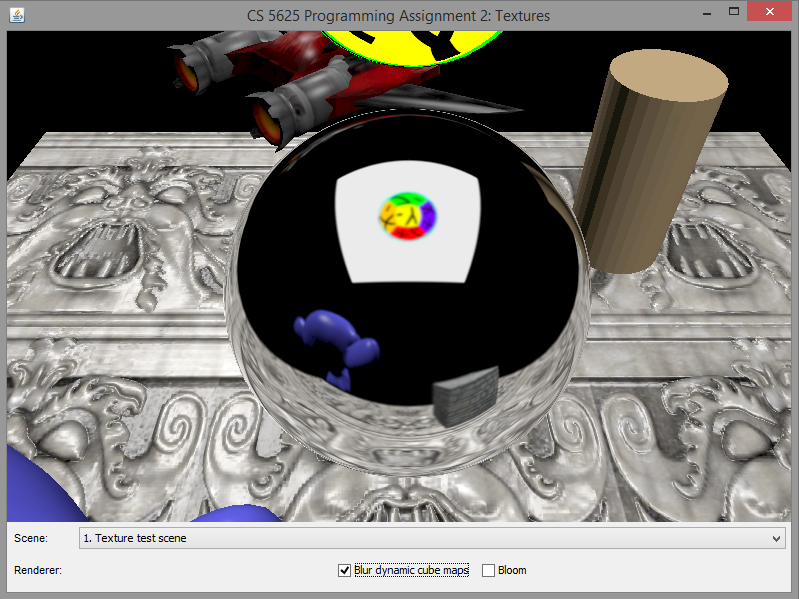No blurring With blurring

Edit:

to renders the bloom effect when enabled.

The bloom is a visual effect that aims to simulate the phenomenon in which imperfect camera lenses produces halos of light around bright pixels While there is a physical basis for the effect, we will be simulating it in a completely ad hoc way as follows.

First, we only keep pixels that are "brighter" than a certain threshold. Here, the brightness of a pixel is defined as: $$\mathrm{brightness} = 0.299 r + 0.587g + 0.114b.$$ (See this document for the source of the formula.) The threshold for the brightness value is given in the brightnessThreshold field of ForwardRenderer. The resulting of thresholding is as follows: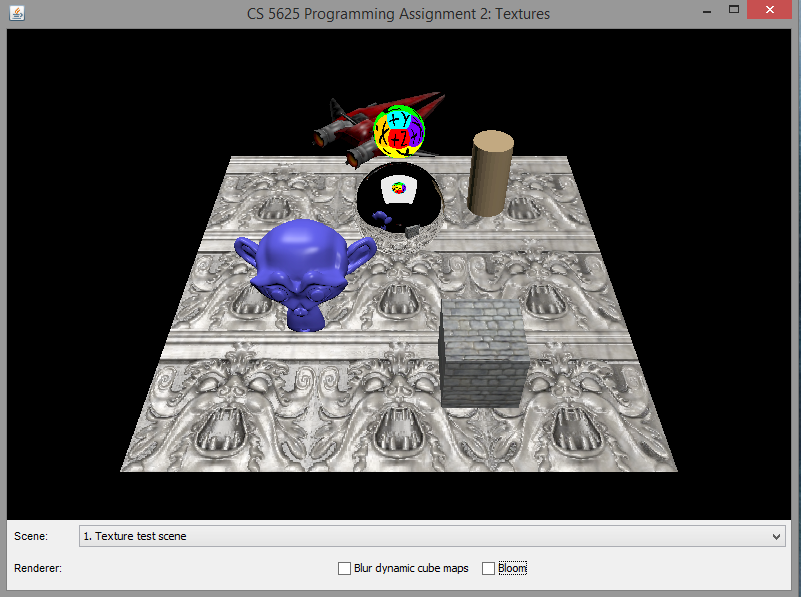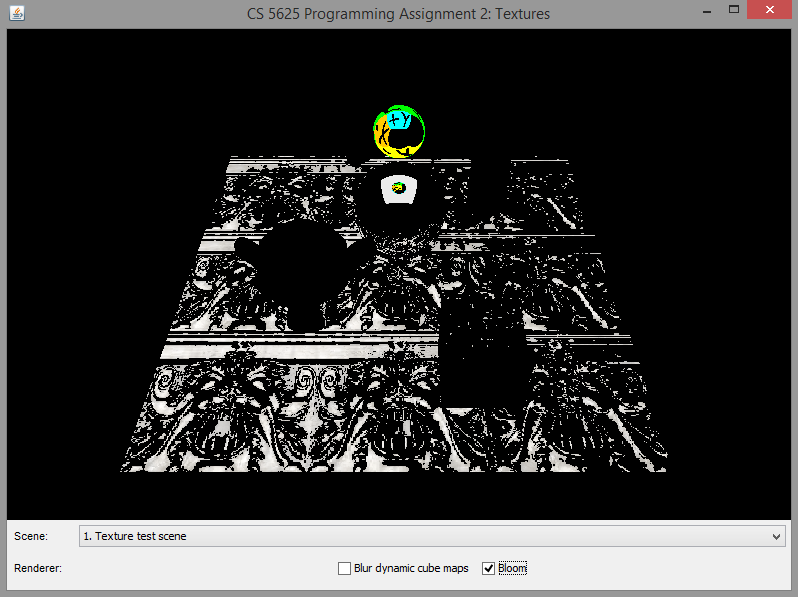Original image After thresholding with threshold 0.5

Then, we convolve the threshold images with 4 Gaussian kernels, each with different width, to blur it. The sizes of the kernel windows and the standard deviation of the kernels are given in the bloomFilterSizes and the bloomFilterStdev fields, respectively. The results of the convolutions are as follows: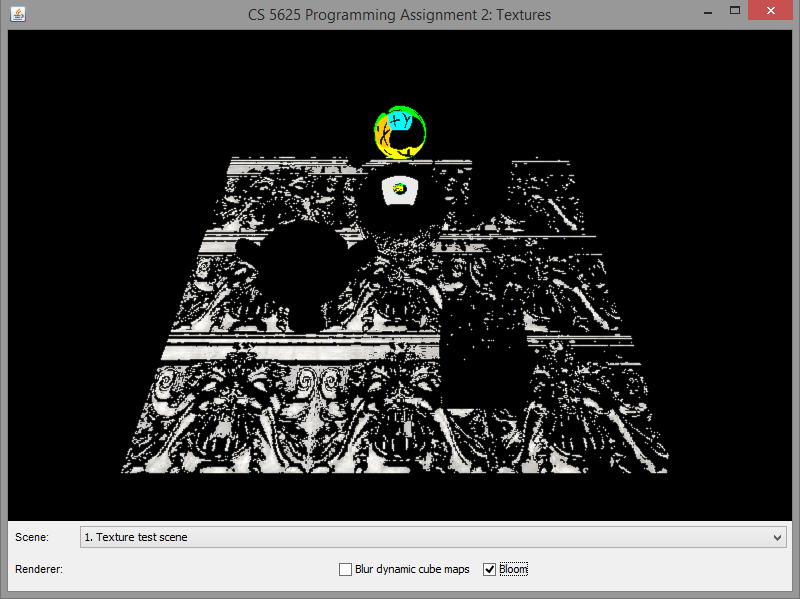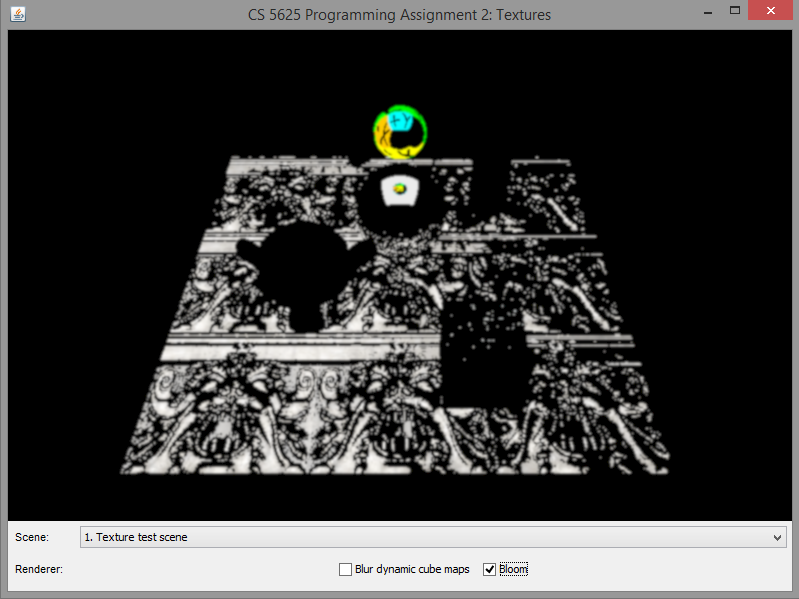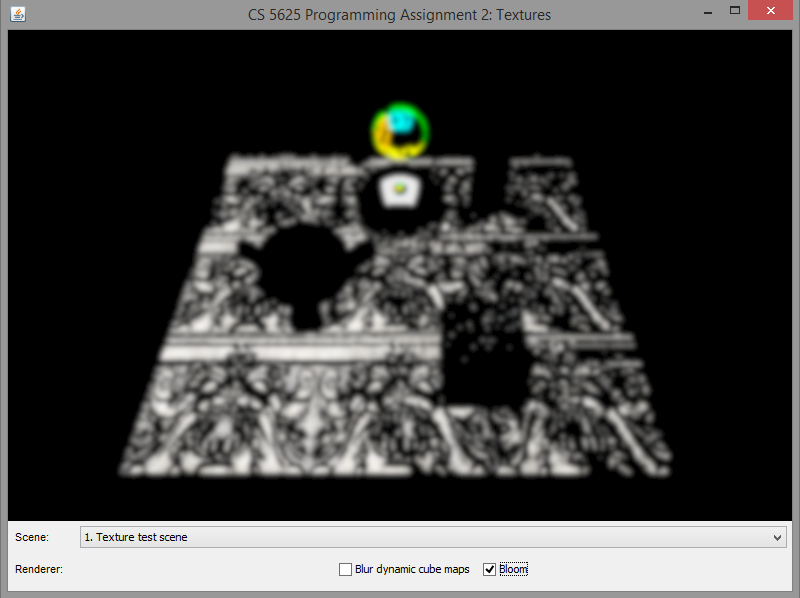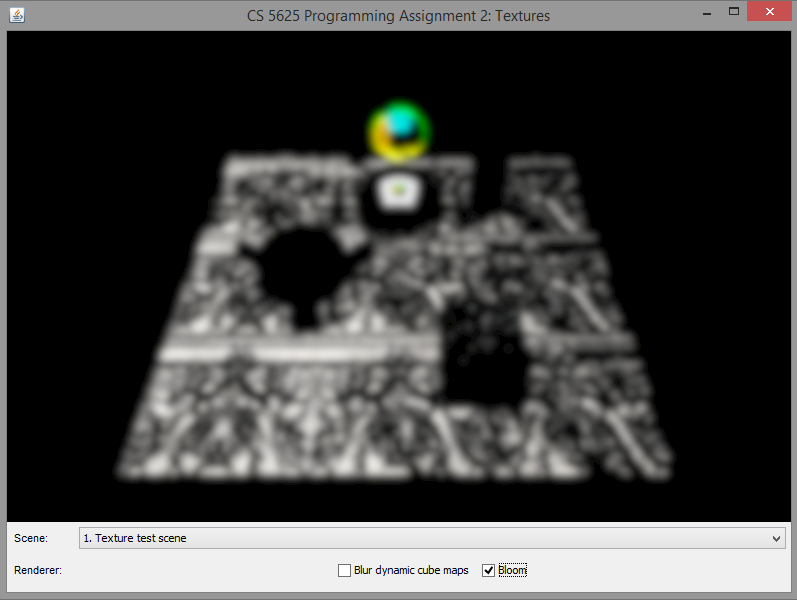Blur #1 Blur #2 Blur #3 Blur #4

We then scale each image by the constants stored in bloomFilterScales array and add the scaled images to the original image to produce the final image. (In our case, the constants are all $1/4$, which basically mean we average the blurred images together.)$+$ $\frac{1}{4}$$+$ $\frac{1}{4}$$+$ $\frac{1}{4}$$+$ $\frac{1}{4}$$=$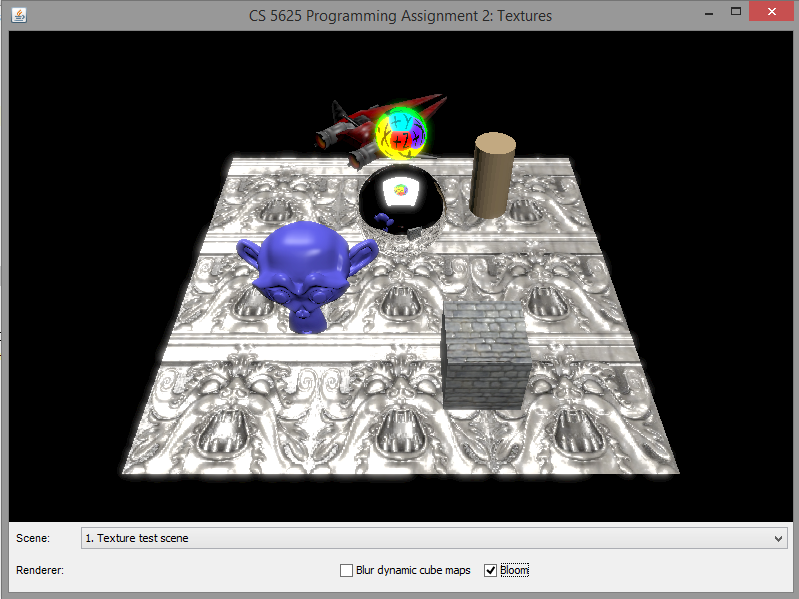Original Blur #1 Blur #2 Blur #3 Blur #4 Final

The images below show the differences between the original and the final image with the bloom effect fully applied:Original image Final image

Note that this task may involve the use of one or more shaders which we do not provide to you. Write your own shaders to get the job done. It also involes multi-step manipulation of the frame buffer objects and textures, and again we leave it to you to figure out how this should be done. You are free to declare new fields in the ForwardRenderer class if needed be. Also, the algorithm we presented here is certainly not the most efficient. You can try the trick in this web page to improve upon it.

Run the cs5625.pa2.PA2_BlendShapes class, and you will see that the program allows you to change between three characters: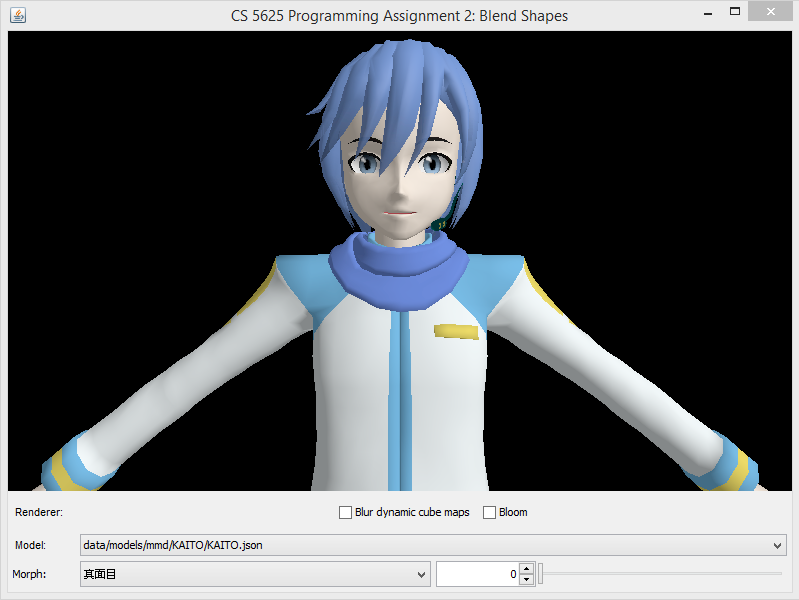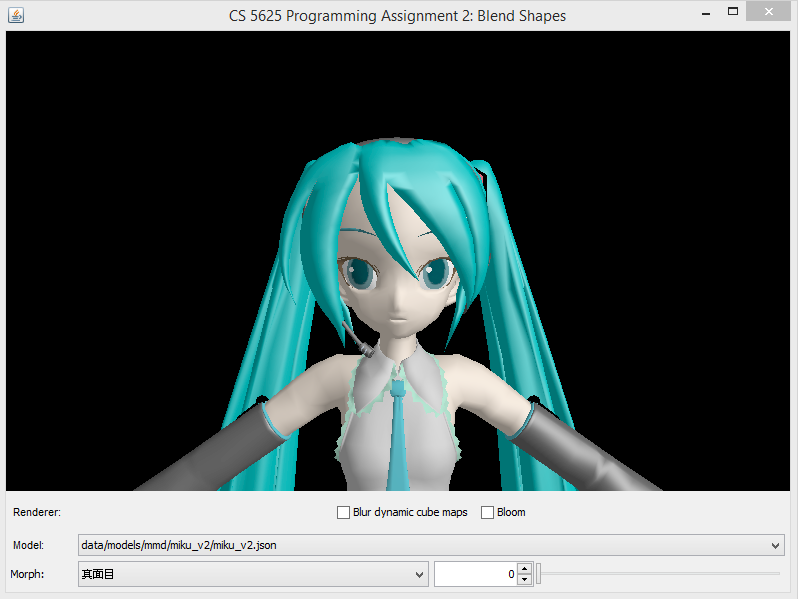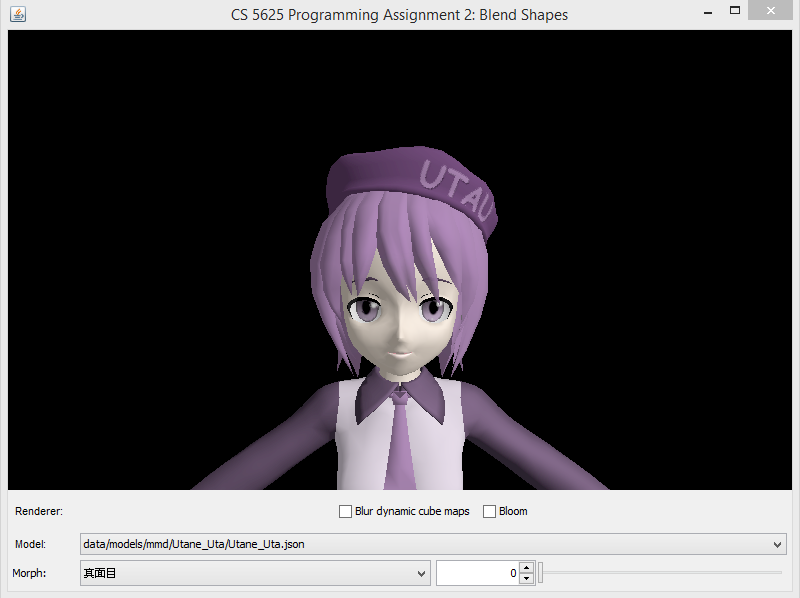KAITO Hatsune Miku Utane Uta

Each charcter has a set of blend shapes or, as we shall call it in this assignment, "morphs," associated with it. A morph in this assigment modifies some facial features of the character, making him/her wink, for example. A specific morph can be selected by the combo box next in the bottom row. Each morph also has an associated "weight," which is a floating point number from 0 to 1. You can set this value using the spinner and the slider in the bottom row.

The basics of blend shapes were discussed in CS4620 in the animation lecture (slides 68–69). The idea is that each vertex comes with a 3D position for each of $N$ blend shapes, and the animation is controlled by per-frame weights $w_j$ for each blend shape, which sum to $1$. If $\mathbf{p}_{ij}$ is the position of vertex $i$ in blend shape $j$, and $w_j$ is the weight of blend shape $j$ in the current frame, then the position of vertex $i$ is $$\mathbf{p}_i' = \sum_j w_j\mathbf{p}_{ij}.$$

Because the blend shapes are the same over most of the character, in this assignment the blend shapes are stored as displacements from a neutral pose; that is $$\mathbf{p}_{ij} = \mathbf{p}_i + \mathbf{d}_{ij}$$ so that the blended position is $$\mathbf{p}_i' = \mathbf{p}_i + \sum_j w_j\mathbf{d}_{ij}.$$ Since the neutral shape has all displacements zero, it can be left out, and the weights no longer have to sum to $1$.

These displacements are zero for most vertices in each blend shape, so for each blend shape $j$ the intput file contains a list of the indices $i$ that have nonzero displacements, with values of $\mathbf{d}_{ij}$ just for those vertices. The data is stored in files like student/data/models/mmd/KAITO/KAITO.json; search for the class name SkeletalMeshMorph to see how the data looks. Storing things this way also makes it easy to construct a list of the blend shapes that affect a particular vertex, and to process only those displacements in the vertex shader. The code to read these files and store the data in textures is provided. All this leads to a great decrease in storage and computation, at the cost of somewhat more complex indexing.

The names of the blend shapes for these anime characters are written in Japanese; translations for the character KAITO are in student/data/models/mmd/KAITO/KAITO.names.txt. The names for the other characters are mostly similar, but contributions of missing translations, or translations for the bone names, are welcome! (Just send them to us and we will push them to the repository for everyone.)

so that the shader implement morphing of vertices.

Information about morphs come to the shader in pieces of three data structures.

• The first is the vert_morphWeights texture rectangle. This is a texture of size $(\mbox{number of morphs}) \times 1$ such that the value of the $(j,0)$-pixel contains the weight of the $j$th morph. You can use the getMorphWeight function to fetch the weight of the morph with a given index (which starts from 0).
• The second is the vert_morphDisplacements texture rectangle. You can think of this as an array containing displcements to individual vertices from all the morphs, sorted by the indices of the vertex. (It will become apparent soon why we sort it this way.) You can recover the $i$th entry of the array using the getMorphDisplacementInfo(int i) function. The function returns a vec4 whose x-, y-, and z-components denote the displacement being applied to the vertex position. The w-component stores the index of the morph having this displacement.
• The third is the vertex data. Two relevent attributes are the vert_morphStart and the vert_morphCount attributes. The vert_morphCount indicates the number of morphs the current vertex fall under influences of, and the vert_morphStart indicates the first index into the vert_morphDisplacements array of elements that hold displacements that the vertex is subjected to under various morphs.

Let $n$ denote the value of vert_morphCount. To compute a morphed vertex position, write a loop that starts fetching the vert_morphDisplacements array at indices vert_morphStart, vert_morphStart+1, vert_morphStart+2, $\dotsc$, vert_morphStart+$n$-1. Each of these texture fetching will give you information about the largest displacement that you can apply to the vertex and the index of the associated morph. Now, with the index of the morph at hand, you can find the weight of the morph from the vert_morphWeights texture. Once you know the weight, scale the displacement by the it and add the resulting weighted displacement to the vertex position. (For morphing, you only change the vertex position and do not have to care about the normal, the tangent, or the bitangent.)

A correct implementation of the shader should yield the following images

 Morph KAITO Hatsune Miku Utane Uta まばたき (wink)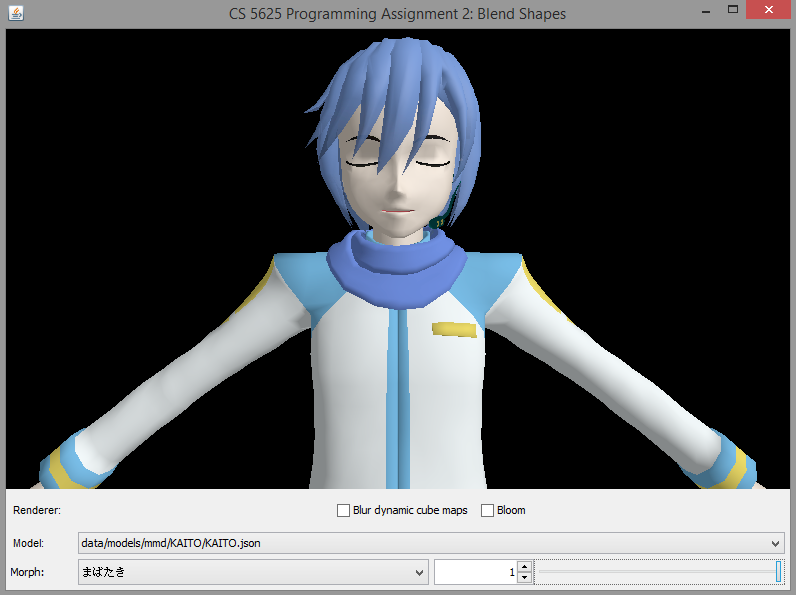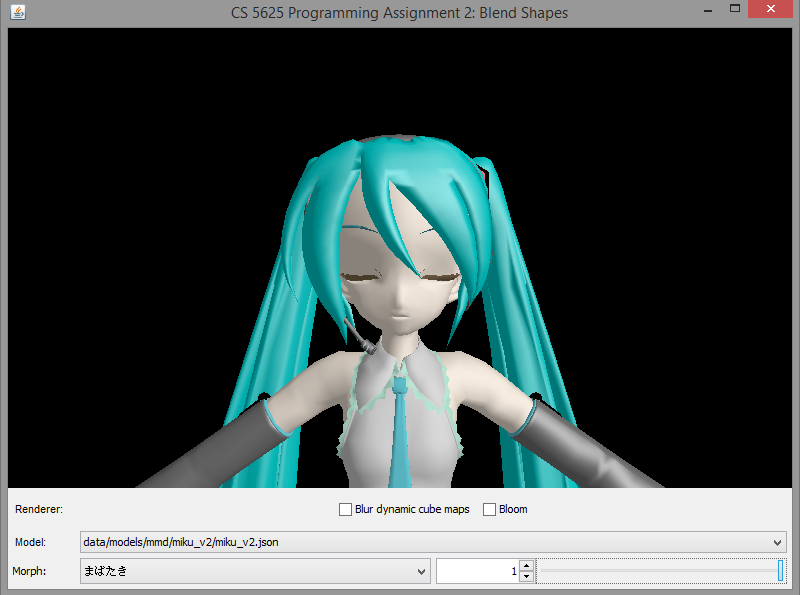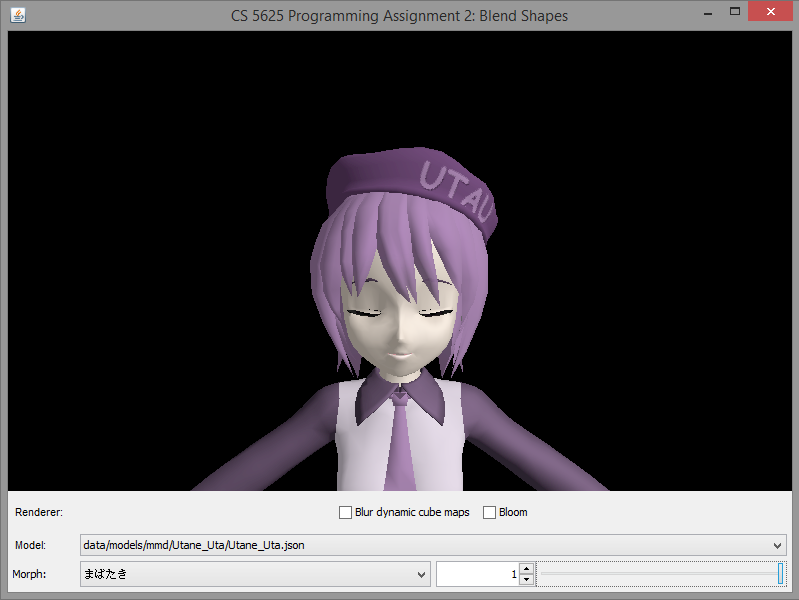あ (saying "ah")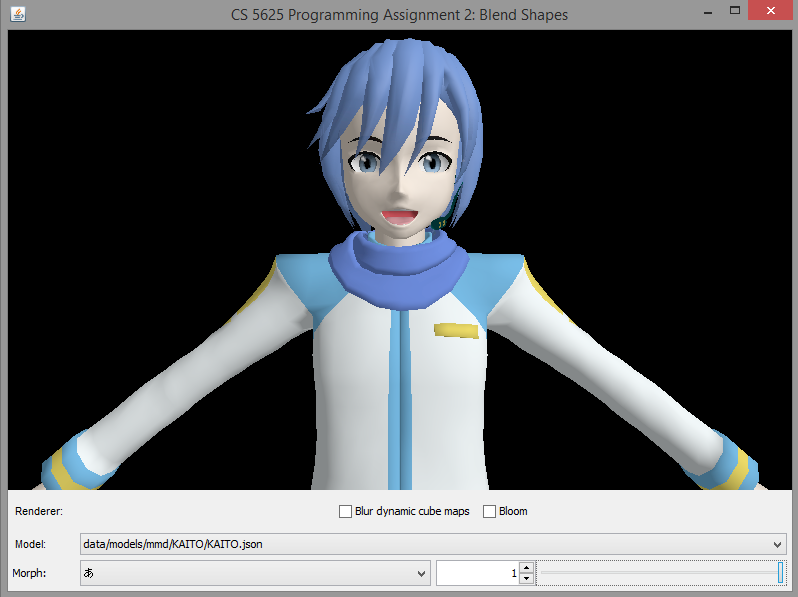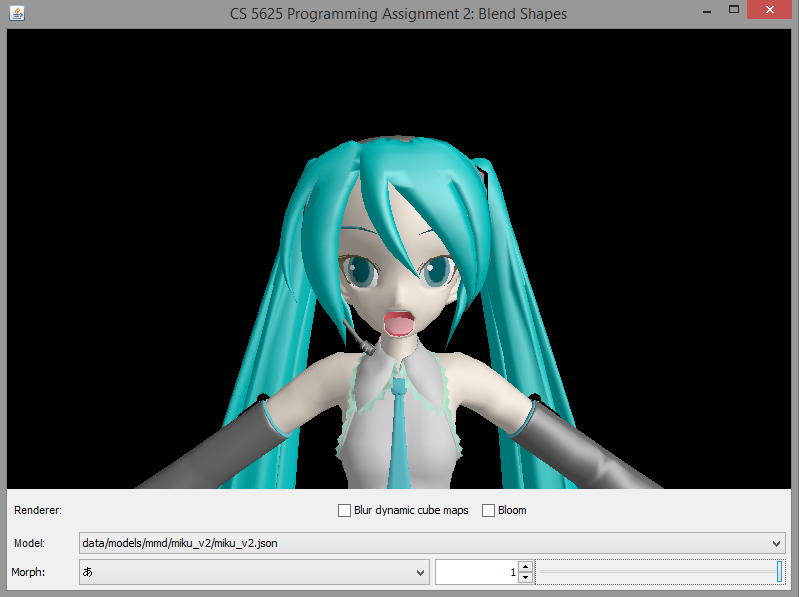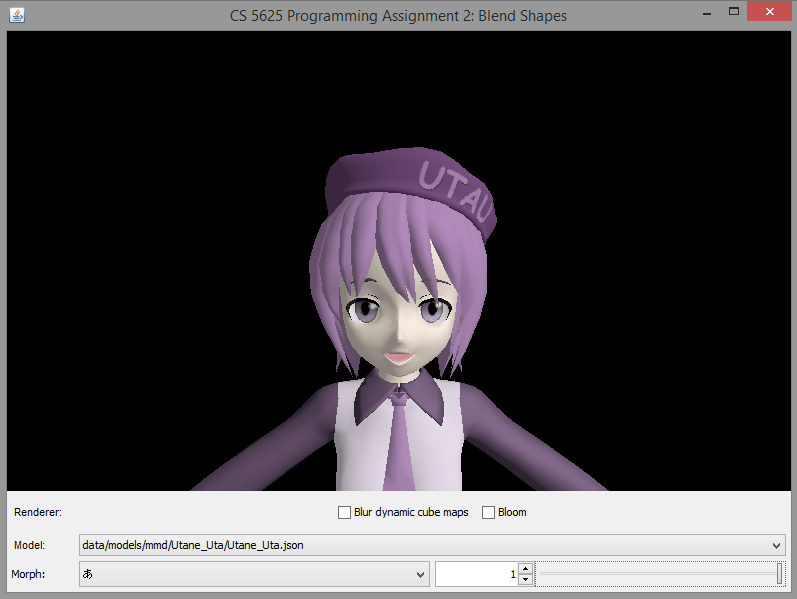瞳小 (small irises)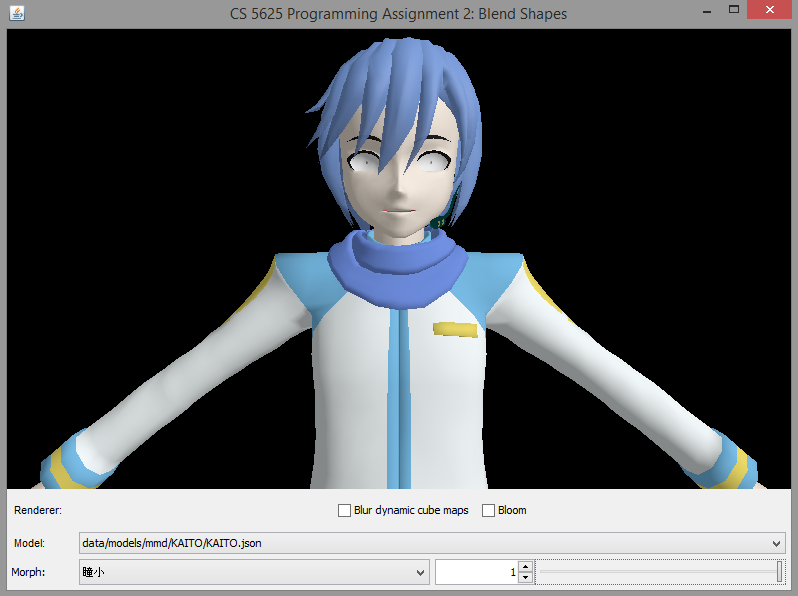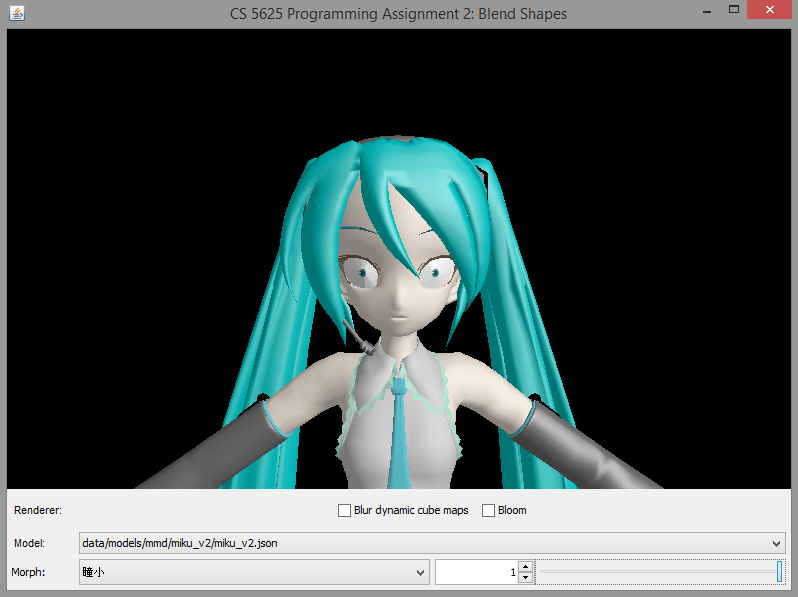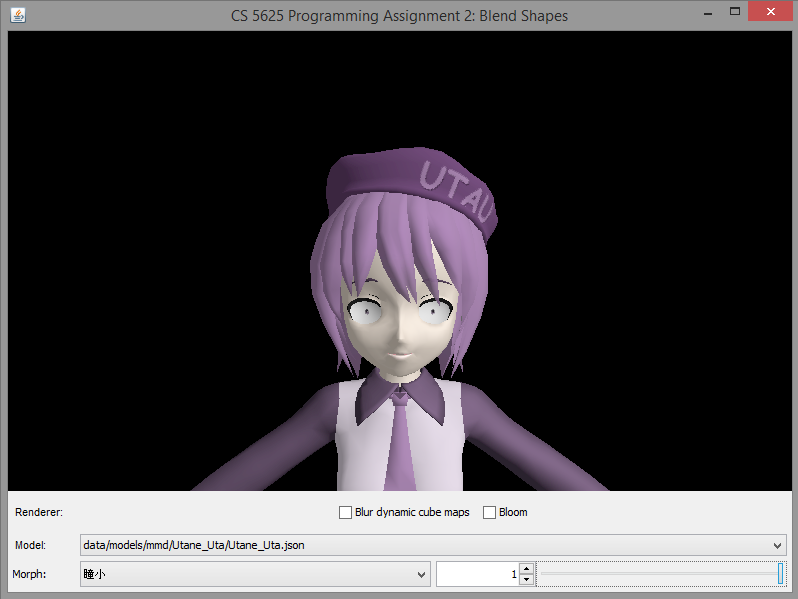## Task 8: Linear blend skinning

The last piece of our character animation system is linear blend skinning, a technique that allows vertices of a mesh to deform according to an underlying skeleton. Linear blend skinning is discussed in slides 62–67 of the CS4620 animation lecture. The basic idea is that the pose of an animated character is specified by using per-frame linear transformations (usually rigid) for each of a set of bones in the skeleton, and the deformed positions of the mesh (the "skin") are computed by applying a combination of the bone transformations to the neutral vertex position. The influence of bone $j$ on vertex $i$ is given by a fixed (not per-frame) weight $w_{ij}$, so the deformed position is: $$\mathbf{p}_i' = \sum_j w_{ij} \mathbf{P}_i.$$ Since each vertex is normally influenced by only a few bones, in this assignment we store the weights for a vertex $i$ by keeping a list of the bone indices $j$ for which the weights $w_{ij}$ are nonzero, togther with the values of those weights. Each vertex is only allowed to be influenced by up to four bones, so we store exactly four indices for each vertex, using the value $-1$ to indicate an unused index. You can see an example of the input data in the file student/data/models/mmd/KAITO/KAITO.json, in the fields boneIndices and boneWeights of the ConcreteSkeletalMesh.

The animation data for each frame consists of a bone transformation for each bone and a morph weight for each blend shape; only nonzero morph weights are stored. See for example the single-frame animation in student/data/motions/KAITO/stand01.json.

to implement it.

The SkeletalMeshPoseManager class manages the "pose" of an articulated mesh. A pose is basically the collection of morph weights (each a floating point number from 0 to 1) and the configurations of skeletal bones (each bone has a corresponding displacement and a rotation). The morph weights are stored in the morphWeights field. The bone displacements and rotations associated with the pose are stored in the boneDisplacements and boneRotations fields, respectively. Morph weight processing has been completely implemented, so you do not need to worry about it. However, you will need to implement the computation of transformation matrices for each bone in this task.

The method updateBoneXformTexture computes for each bone a transformation matrix for use with linear blend skinning and stores the result in the boneXforms field so that the $i$th element stores the matrix for the $i$th bone. The bones themselves are stored in the bones field. Each bone is represented by the SkeletalMeshBone structure, and it has two piece of important information:

• the index of the parent bone (-1 if it has none) in the bones field, and
• the displacement from the child bone to the parent bone in rest position. (If the bone has no parent, it is the world space position of the bone.)

We now discuss the computation of the matrices for linear blend skinning. Let:

• $T_i^{\mathrm{R}}$ denote the 4x4 translation matrix associated with the displacement of Bone $i$ to its parent in rest position.
• $T_i^{\mathrm{P}}$ denote the 4x4 translation matrix associated with the displacement stored in the $i$th element of boneDisplacements.
• $R_i^{\mathrm{P}}$ denote the 4x4 rotation matrix associated with the quaternion stored in the $i$th element of boneRotations.
Now, let us say that we are interested in Bone $i_k$, which is a child of Bone $i_{k-1}$, which in turn is a child of Bone $i_{k-2}$, and so on until we reach bone $i_0$ which does not have a parent. The matrix for linear blend skinning can be computed in two steps:
1. First, compute the 4x4 transformation matrix associated with the translation from the origin to the world position of Bone $i_k$ in rest position. This matrix is given by: $$M^{\mathrm{R}}_{i_k} = T^{\mathrm{R}}_{i_0} T^{\mathrm{R}}_{i_1} T^{\mathrm{R}}_{i_2} \dotsm T^{\mathrm{R}}_{i_{k-1}} T^{\mathrm{R}}_{i_k}.$$
2. Second, compute the 4x4 transformation matrix associated with going from the origin to the world position of Bone $i_k$ according to the pose. This matrix is given by: $$M^{\mathrm{P}}_{i_k} = ( T^{\mathrm{R}}_{i_0} T^{\mathrm{P}}_{i_0} R^{\mathrm{P}}_{i_0} ) ( T^{\mathrm{R}}_{i_1} T^{\mathrm{P}}_{i_1} R^{\mathrm{P}}_{i_1} ) \dotsm ( T^{\mathrm{R}}_{i_{k-1}} T_{i_{k-1}}^{\mathrm{P}} R_{i_{k-1}}^{\mathrm{P}} ) ( T^{\mathrm{R}}_{i_k} T_{i_k}^{\mathrm{P}} R_{i_k}^{\mathrm{P}} ).$$
3. The bone matrix for linear blend skinning is then given by: $$M_{i_k} = M^{\mathrm{P}}_{i_k} ( M^{\mathrm{R}}_{i_k} )^{-1}.$$

After you have computed the matrices, the rest of the updateBoneXformTexture will take care of sending the information to the shader for you. The next part of this task is to write the shader to make use of this information. In skeletal_mesh.vert, we have provided the getBoneXform function such that calling getBoneXform(i) fetches the matrix boneXforms.get(i) that you previously computed.

To perform linear blend skinning, we first morph the vertex according to the blend shapes as was done in the last task. Let us denote the morphed vertex position by $\mathbf{p}$. The vertex can be influenced by a number of bones, and each of this bone has different amount of influence on the vertex, indicated by the bone's weight. The indices of the bones that influence the vertex is stored in the vert_boneIndices attributes, a vec4, meaning that there can be at most four bones that can influence the vertex. You can retrieve the index of the $j$th bone with the expression vert_boneIndices[j] where $j$ ranges from 0 to 3. Some of these indices may be -1, indicating that this is not a valid bone and should be ignored. The weights of the bones are stored in the attribute vert_boneWeights, and the weight of the $j$th bone can be retrieved by the expression vert_boneWeights[j]. Now, suppose that the indices of the bones are $j_0$, $j_1$, $j_2$, and $j_3$, and the associated weights are $w_{j_0}$, $w_{j_1}$, $w_{j_2}$, and $w_{j_3}$. Then, the linear blended vertex position is given by: $$\mbox{blended vertex position} = w_{j_0} M_{j_0} p + w_{j_1} M_{j_1} p + w_{j_2} M_{j_2} p + w_{j_3} M_{j_3} p.$$ Now, you also have to compute the blended tangent, bitangent, and normal. We suggest that you compute the tangent and bitangent using the formula similar to the above. Then, you can compute the normal by computing the cross product between the two vectors.

Lastly, note that the positions and tangent frame you computed in the last paragraph are in object space. You have to transform them with the appropriate matrices to pass them to the fragment shaders and the rasterization unit. However, the code to do this has already been provided for you in the shader.

You can test the full implementation of the character animation system by running the cs5625.pa2.PA2_Animations class. Here, you still have the option of selecting among the three characters, but you can also subject them to 5 poses/animations. A correct implementation of the system should produce the following images:

 Animation/Pose KAITO Hatsune Miku Utane Uta Stand 1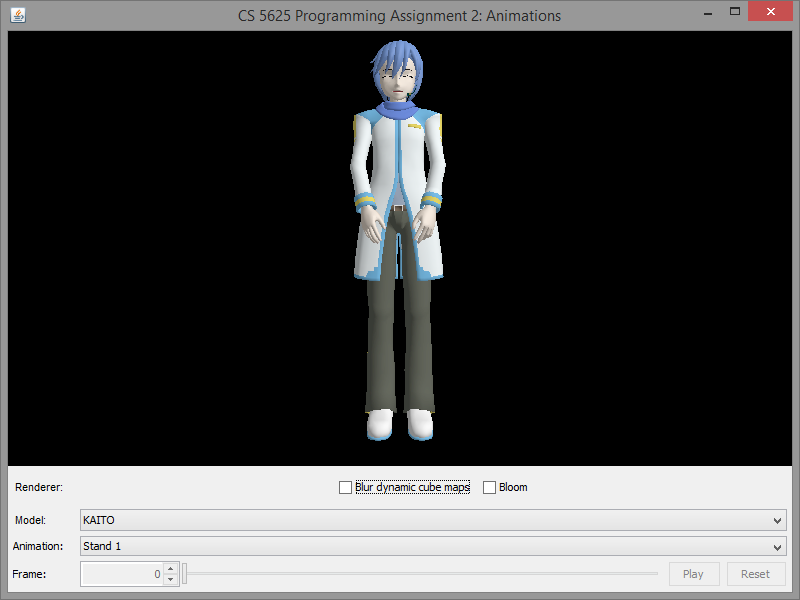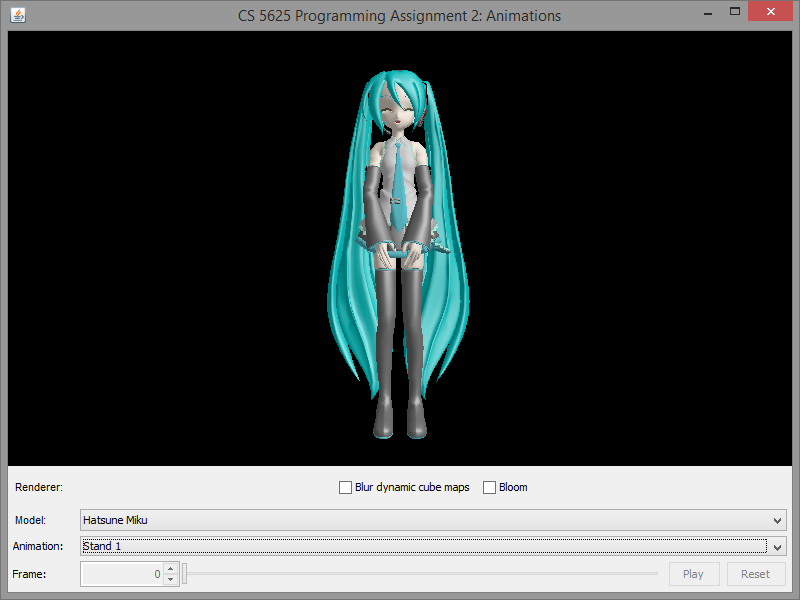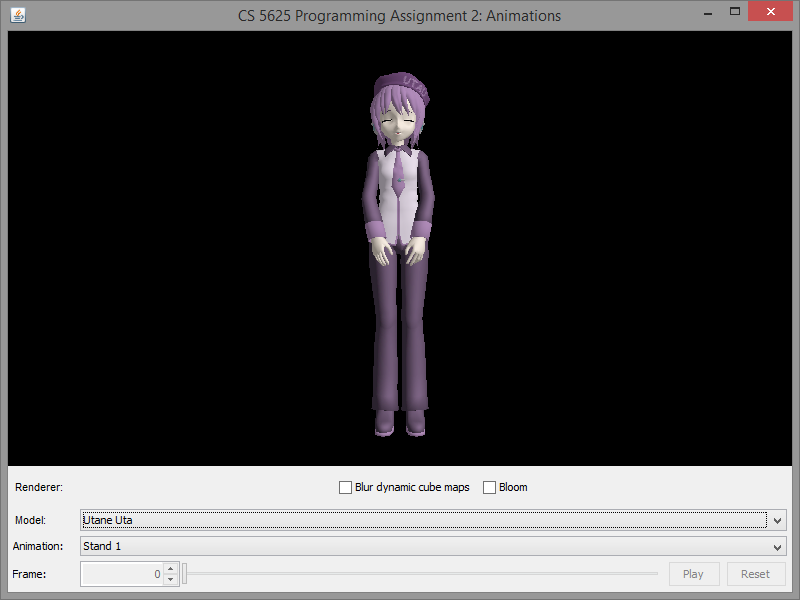Stand 2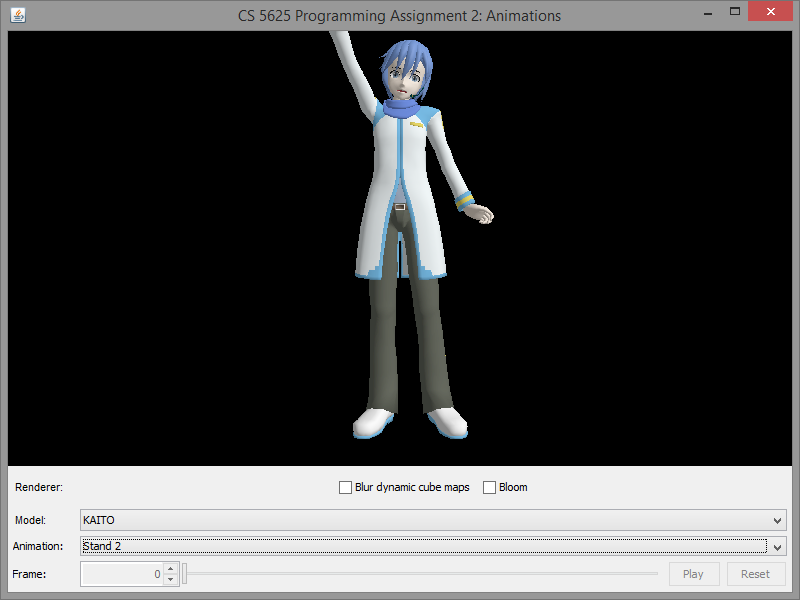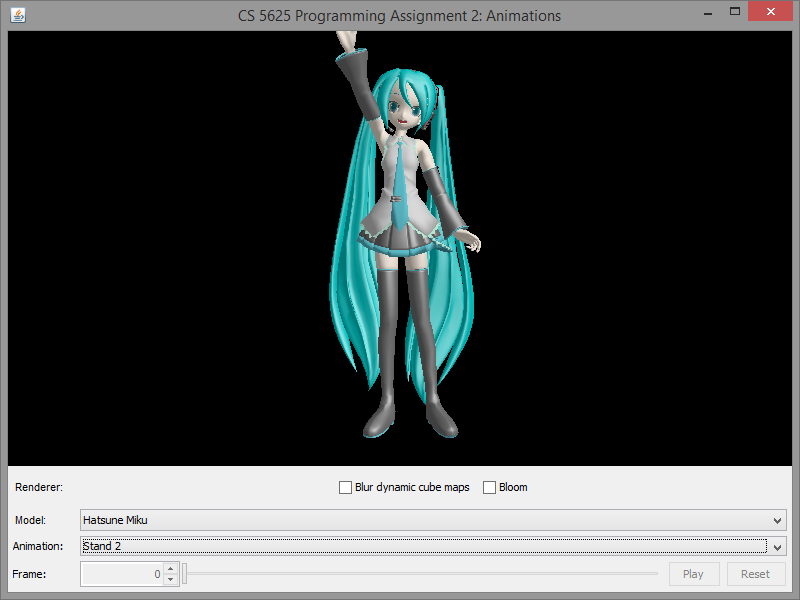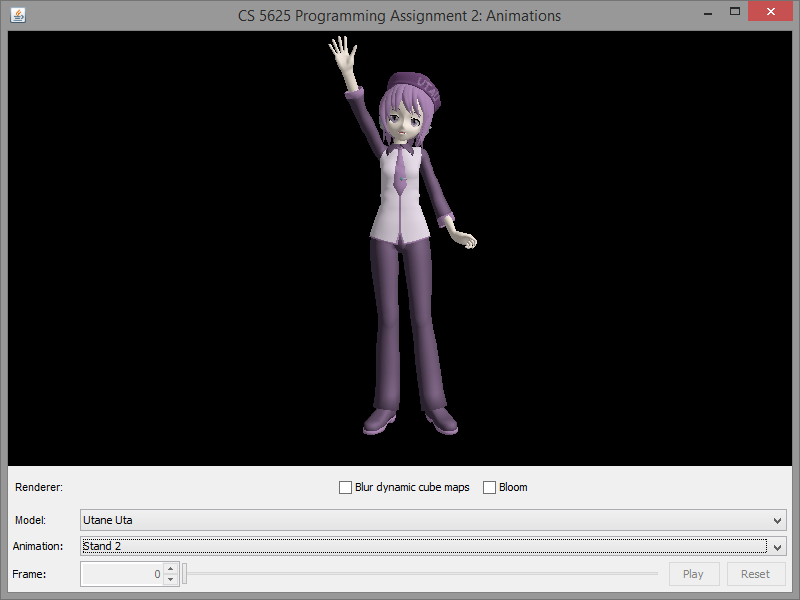Stand 3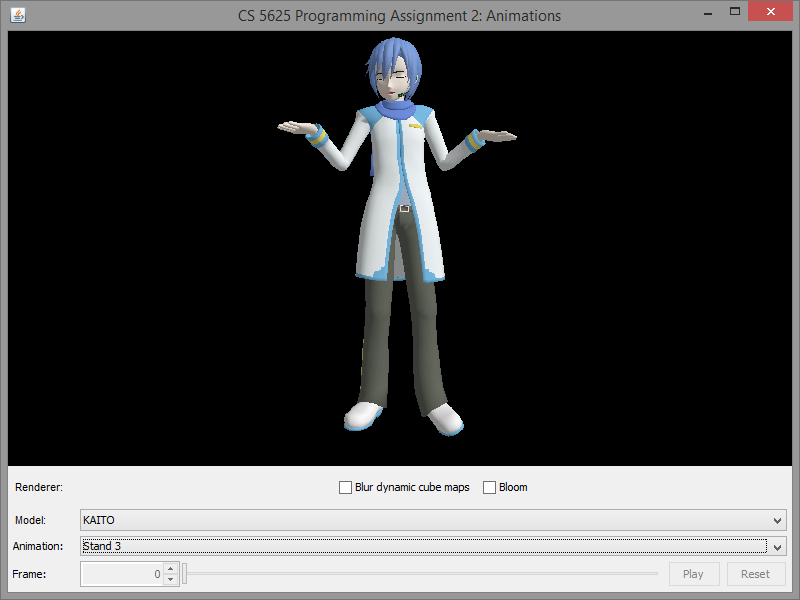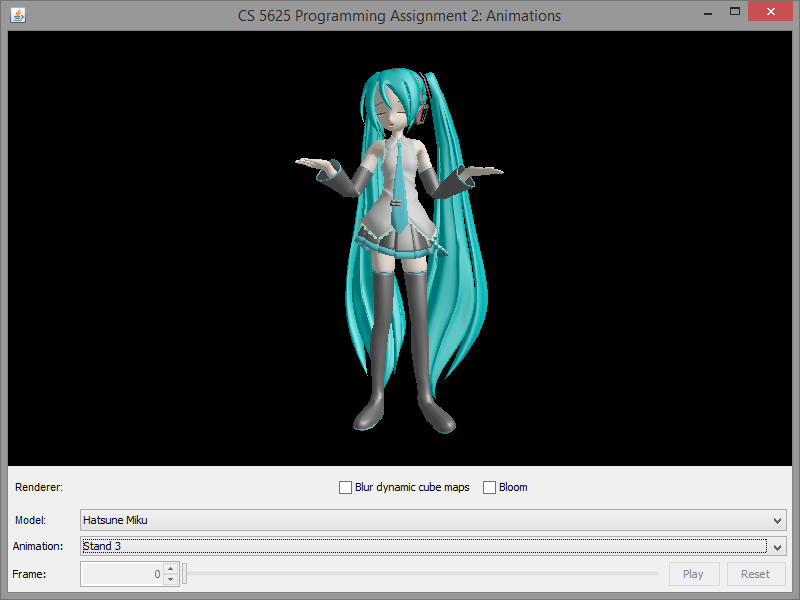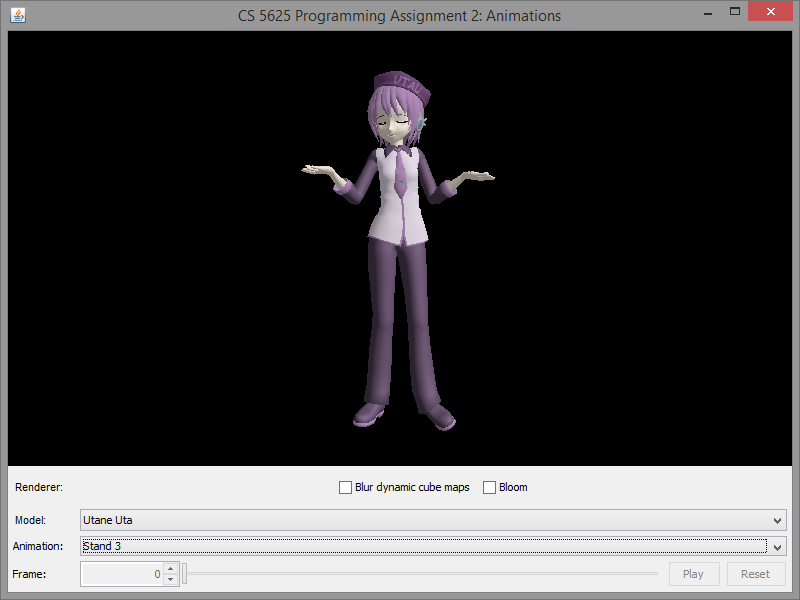Suki Yuki Maji Magic! (frame 3,000)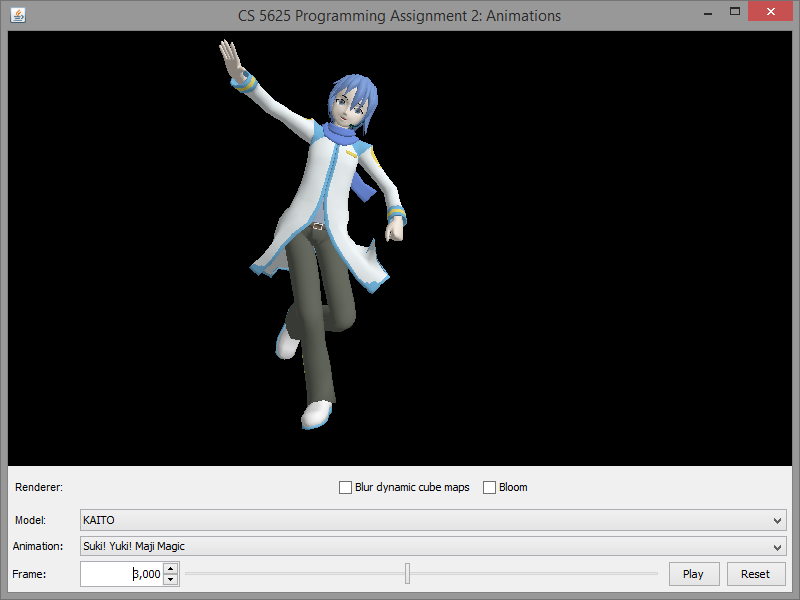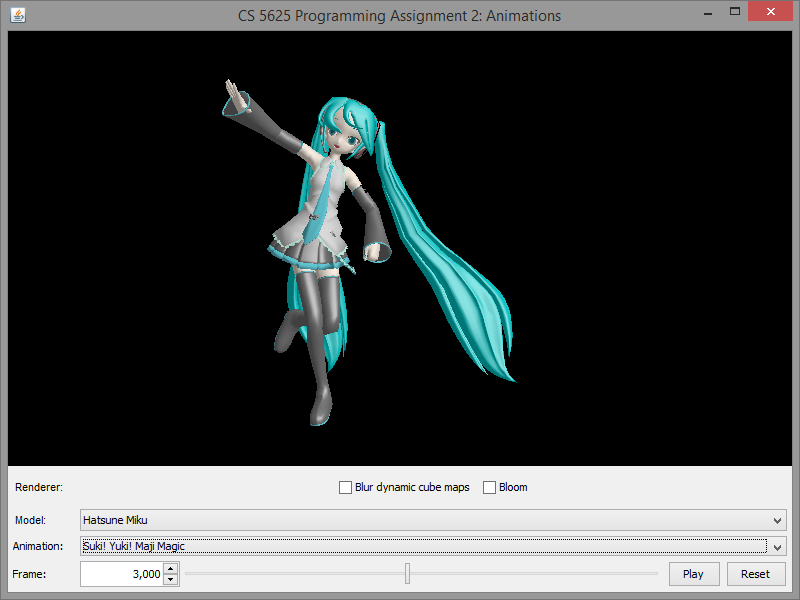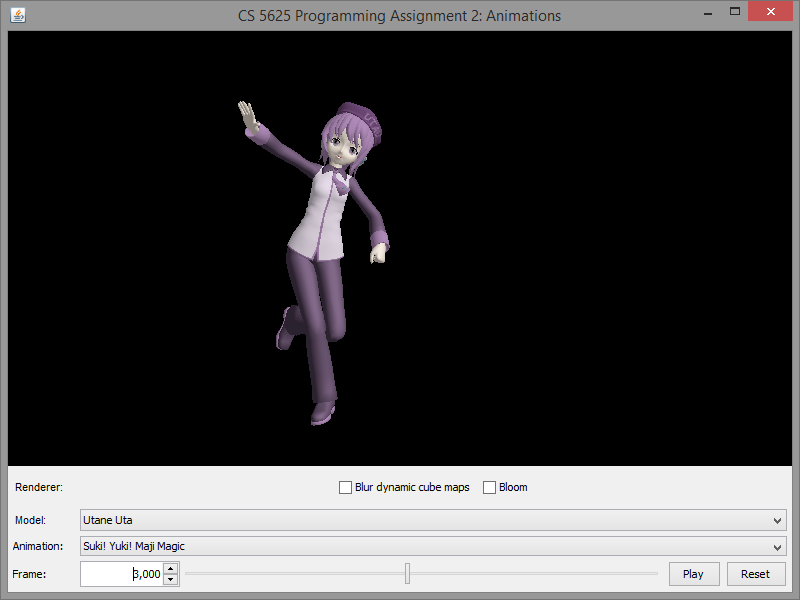Neko Mimi Switch (frame 3,000)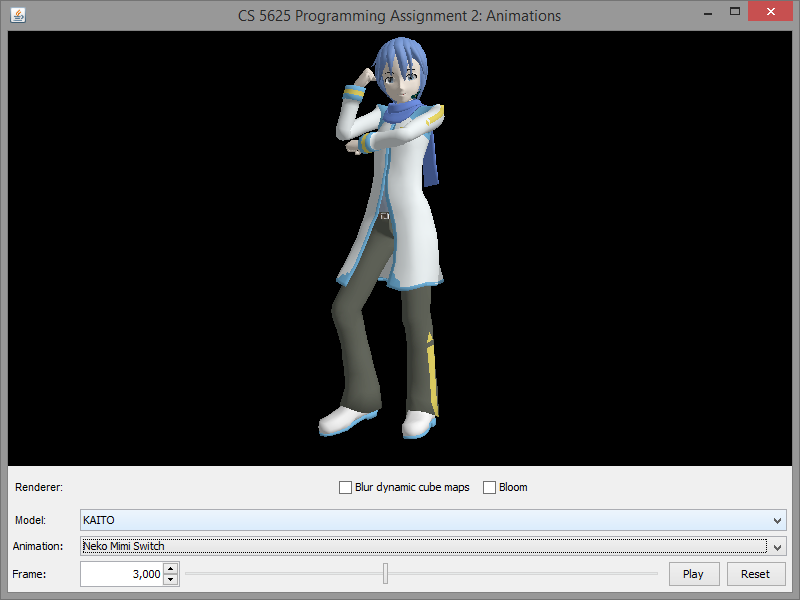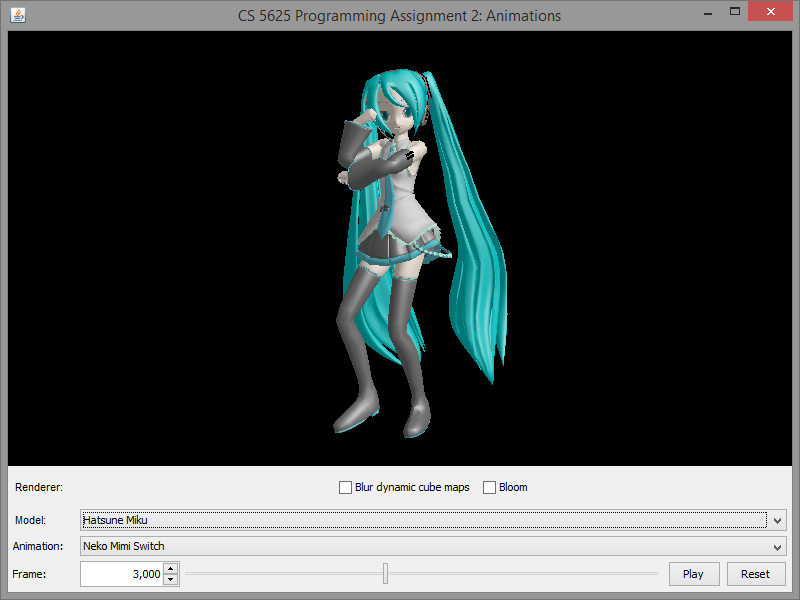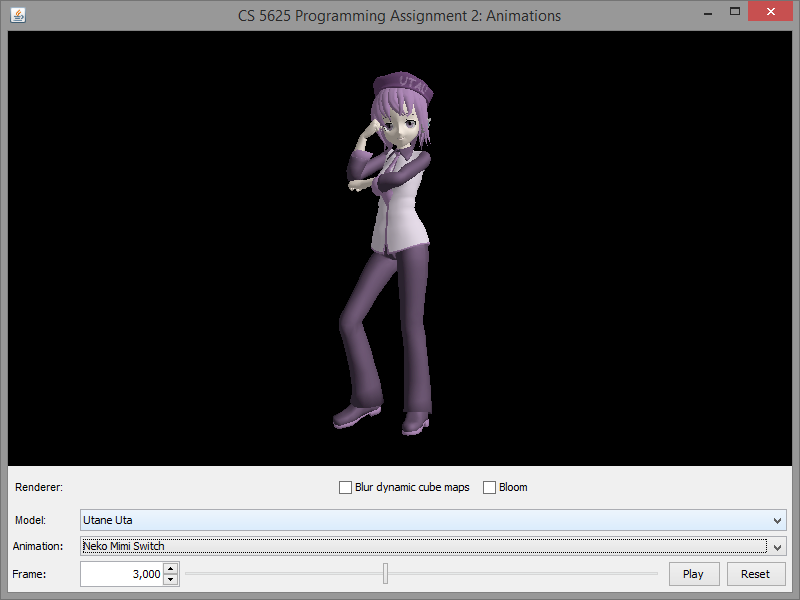For your reference, see the following Youtube videos for the full Suki Yuki Maji Magic! and Neko Mimi Switch motions.